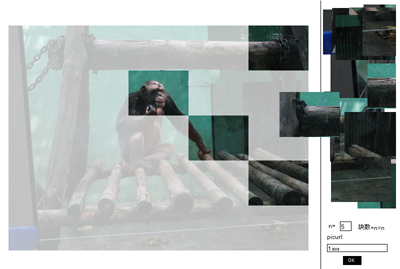## [AS3][开源]拼图游戏。。终于完成。。

2007-8-14 评论(5) 分类:作品 Tags:## [AS3]摄像头录制

2007-7-30 评论(1) 分类:作品 Tags:

AS文件代码：

package{
import flash.display.Sprite
import flash.media.Camera
import flash.events.MouseEvent
import flash.display.BitmapData
import flash.events.Event
import flash.media.Video
import flash.display.Bitmap
public class camera extends Sprite{
var mycamera:Camera=Camera.getCamera()
var myvideo:Video=new Video()
var bit:Array
var vwidth=250
var vheight=200
var i:uint=1
var playvideo:Bitmap=new Bitmap()
public function camera (){
myvideo.attachCamera(mycamera)
myvideo.smoothing=true
myvideo.width=vwidth
myvideo.height=vheight
myvideo.x=50
myvideo.y=30
}
public function rec_btn(btn){
}
public function play_btn(btn){
}
public function stop_btn(btn){
}
public function on_rec_btn(event:MouseEvent){
bit=new Array()
i=1
}
public function on_stop_btn(event:MouseEvent){
removeEventListener(Event.ENTER_FRAME,onenterframe)
removeEventListener(Event.ENTER_FRAME,onenterframeplay)

}
public function on_play_btn(event:MouseEvent){
}
private function onenterframe(event:Event){
var rec:BitmapData=new BitmapData(vwidth,vheight,true,0xff)
rec.draw(myvideo)
bit.push(rec)
}
private function onenterframeplay(event:Event){
if(i<bit.length){
playvideo=new Bitmap(bit[i])
playvideo.x=360
playvideo.y=30
i++
}else{
removeEventListener(Event.ENTER_FRAME,onenterframeplay)
}

}
}
}

## [AS3]输入两边一角画三角形

2007-7-29 评论(0) 分类:作品 Tags:

package{
import flash.display.Sprite
import flash.events.MouseEvent
import flash.text.TextField
import flash.text.TextFieldType
public class triangle extends Sprite{
private var btnS:Sprite=new Sprite()
private var drawS:Sprite=new Sprite()
var aside:TextField=createtxt(150,350,40,20)
var bside:TextField=createtxt(200,350,40,20)
var angle:TextField=createtxt(250,350,40,20)
var ctrl:TextField=createtxt(350,350,40,20)
public function triangle(){
aside.type=TextFieldType.INPUT
bside.type=TextFieldType.INPUT
angle.type=TextFieldType.INPUT
aside.text=”边长a”
bside.text=”边长b”
angle.text=”ab夹角”
btnS.graphics.beginFill(0x000000)
btnS.graphics.drawRect(300,350,40,20)
btnS.buttonMode=true
}
private function createtxt (x:Number,y:Number,width:Number,height:Number):TextField{
var result:TextField=new TextField()
result.x=x
result.y=y
result.width=width
result.height=height
result.border=true
return result
}
private function onbtnDown(event:MouseEvent){
drawS.graphics.clear()
var a=Number(aside.text)
var b=Number(bside.text)
var ag=Number(angle.text)
if(ag<180){
drawS.graphics.lineStyle(1)
drawS.graphics.moveTo(100,50)
drawS.graphics.lineTo(100+a,50)
drawS.graphics.lineTo(100+a-b*Math.cos(ag/180*Math.PI),50+b*Math.sin(ag/180*Math.PI))
drawS.graphics.lineTo(100,50)
}else{
ctrl.text=”error”
}

}
}
}

## [AS3]使用stage出错的问题。

2007-7-29 评论(0) 分类:技术文章 Tags:

1、文档类的构造函数中可以直接使用stage属性
2、非文档类可以通过参数传递到类里面。
class Test extends Shape
{
function Test(stage:Stage)
{
}
}
3、不想传递参数时，要注意代码的顺序。
class Test extends Shape
{
function Test()
{
}
functon useStage()
{
trace(stage)
}
}

var test=new Test()
test.useStage()//null，你的问题出在这里
test.useStage()//[object Stage]

## 输入角度画三角形

2007-7-26 评论(0) 分类:作品 Tags:

_root.createEmptyMovieClip(“line_mc”,100)
ok_btn.onRelease=function(){
lineang1=-(ang1/180)*Math.PI
lineang2=(ang2/180)*Math.PI
line_mc.clear()
line_mc.lineStyle(1)
line_mc.moveTo(50,50)
line_mc.lineTo(200,50)
line_mc.beginFill(0xFF99CC);
line_mc.moveTo(200,50)
line_mc.lineTo(200-300*Math.cos(lineang1),50-300*Math.sin(lineang1))
line_mc.moveTo(50,50)
line_mc.lineTo(50+300*Math.cos(lineang2),50+300*Math.sin(lineang2))
line_mc.endFill();
}

FLA源文件

## 求定积分的FLASH

2006-6-4 评论(0) 分类:作品 Tags:

## [FLASH程序]单摆

2006-4-10 评论(0) 分类:作品 Tags:

## NBA图片程序

2006-3-5 评论(0) 分类:作品 Tags:

NBA图片程序完成了~~因为程序比较容易，所以写代码的时间比较短，很多时间都用在美化和细节上。这次的AS写得很整洁，很清晰，不再像以前那样乱了~~自我感觉很不错~~

## 图片FLASH

2006-3-1 评论(0) 分类:生活 Tags:

## 很久以前在闪吧发的练习。

2006-2-26 评论(1) 分类:技术文章 Tags:

xx=1
a=550/2
b=400/2
r=100
_root.createEmptyMovieClip(“mc”,1)
with(mc){
moveTo(a+r,b)
lineStyle(1)
}
onEnterFrame=function(){
x=a+Math.cos(xx*Math.PI/180)*r//（PS.我到高二开学才知道为什么用三角函数能画出圆，原来是圆的参数方程～～）
y=b+Math.sin(xx*Math.PI/180)*r
mc.lineTo(x,y)
xx+=123//此处若是xx+=1的话就是典型的画圆~+120是正三角形,让它+123是为了在画三角形的时候有所偏转以至达到效果。
}

xx=1
yy=1

a=550/2
b=400/2
r1=100
r2=100

_root.createEmptyMovieClip(“mc”,1)
with(mc){
moveTo(a+r1,b)
lineStyle(1)
}
onEnterFrame=function(){
x=a+Math.cos(xx*Math.PI/180)*r1
y=b+Math.sin(yy*Math.PI/180)*r2
mc.lineTo(x,y)
xx+=123
yy+=123
r1=Math.sin(xx*Math.PI/180)*100
r2=100

}